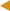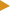﻿ Manipulating real numbers : OnixS .NET Framework FIX EngineFIX Messaging Table of Content Message ValidationManipulating real numbers

This topic depicts issues, related to using real numbers while manipulating FIX messages, as well as solutions for the issues, are offered by the FIX Engine.Double Transformation Issues

FIX Protocol supposes FIX messages that exchange using 'tag=value' presentation. Except for special cases, the field value is a text-based, so regular presentation of real numbers with a decimal point is used: 35=x|268=3|..|270=9462.50|271=5

The interface of the Message class that encapsulates primary operations over FIX fields for a FIX message, offers a few overloads, allowing users to set/get field values of native language types like int, long and double. The field value set/get function-member converts the value of a primitive type into/from its string presentation using natural transformation.

However, for native types like float and double, there's no unambiguous transformation available. That's because double values usually have no finite presentation due to the limitation of the data type. In particular, assume the following initialization of a variable of double type is executed:

C#
`const double Price = 100.10;`
In spite of exact constant definition, the real value, hold by a defined variable, will look like 100.099999999999998, but not 100.1. This is because each double value is expressed as X * 2 ^ Y, where X is mantissa and Y is 2-basis exponent. As a result, there may be transformation issues. For example, when you use the GetDouble(Int32) method to convert a double value from its string presentation which cannot be represented exactly as a 64-bit floating point number (double), you will get the 'cannot cast field value' exception.Using Decimal Instead of double

In contrast to a double type, Decimal presents real numbers use 10-basis exponent. Therefore, each number looks like X * 10 ^ Y, where X is mantissa and Y is an exponent. Parsing Decimal from the text presentation is also free of approximation issues in bounds of values, operated in FIX messages. Thus, operating prices and quantities as Decimal, instead of double types, eliminate issues that are caused by an inexact value presentation, as well as gives performance benefits.